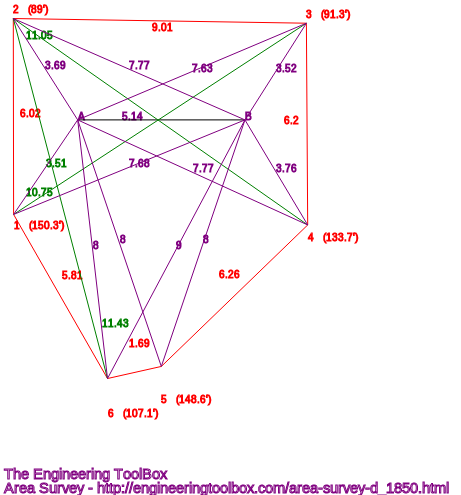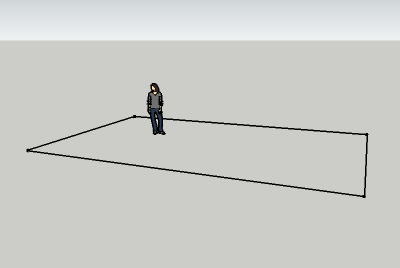Engineering ToolBox - Resources, Tools and Basic Information for Engineering and Design of Technical Applications!

# Area Survey App

## Online app that can be used to make an exact plot of a surveyed area - like a room, a property or any 2D shape.

Use this application to make an exact plot - as indicated below - of a room, a property or any two dimensional area. The plot can be printed or saved with the calculated lengths, diagonales and angles. The plot can also be exported as an area to the amazing free 3D modeling software Sketchup

This application can be very useful if you want to make an exact plot of an "irregular" area with many corners and distorted angles.### How to Use

1. Make the reference line A - B. It can be a virtual line between two points in the same level - or a physical line like the edge of a carpet, the edge of table or kitchen worktop. All points for measure must be visible and possible to measure from both A and B. It is important that the reference line and all measure lines are in the same level (or plane) to avoid plot distortion.
2. Measure the distance A - B. The calculator is generic so you can use any unit  - as long as you use the same unit for all data.
3. Mark the measure points clockwise from 1 to max 9.
4. Measure distances from control points to measure points. Indicate the position of the measured point to the reference line A-B - mark if point is below the reference line.
5. Calculate! - Side lengths, diagonals and angles are calculated and the figure below redraws. Values for export to Sketchup and the Engineering ToolBox extension - "Lines made from Custom Points" are calculated too - see below.

### The Survey Plot

The survey plot below is redrawn when values below are changed and "Calculate!" button is clicked.

• control line A - B is in black
• measured lines are in purple
• calculated sides and angles are in red
• calculated diagonals are in green

### Distance between Control Points

 Distance between Control Points (m, mm, ft, in ...) A - B

### Distance between Control Points and Measured Points

 Distance between Control Points and Measure Points (m, mm, ft, in ...) A B Below A - B 1 2 3 4 5 6 7 8 9

### Export the Plot to Sketchup - the amazing free 3D modelling softwareCopy the red, green and blue coordinates from the textbox below and paste them into the input box in the free Engineering ToolBox extension - "Lines made from Custom Points".

Note! the Engineering ToolBox plugin window must be opened from within Sketchup before pasting the data.

 x, y, z

The values above are plain Cartesian x,y (and z) coordinates that can be transferred manually to almost any type of CAD system.

Tip! In Sketchup - add a "face" to the plot area and use the push/pull tool to make a 3D model of the room or object you are surveying.

### SVG File

The svg file format can be used with most drawing, cad and web based applications.

Copy the SVG code from the window that opens below and paste it to your drawing or document. Note - due to the svg format all coordinates are made positive. Coordinates are offset with the smallest x and y values.

## Related Topics

• ### Drawing Tools

2D and 3D engineering drawing tools.
• ### Mathematics

Mathematical rules and laws - numbers, areas, volumes, exponents, trigonometric functions and more.
• ### Miscellaneous

Engineering related topics like Beaufort Wind Scale, CE-marking, drawing standards and more.

## Related Documents

• ### Area Units Converter

Convert between units of area.
• ### Builder's to Surveyor's Measurements

Convert builder's measurements to surveyor's measurements.
• ### Circles within a Rectangle - Calculator

Calculate the maximum number of circles within a rectangle - can be used to calculate the numbers of pipes or wires in a conduit or similar.
• ### Distance between 3D Points

Distance between two points in a three dimension x, y and z coordinate system - online calculator.
• ### Drawing Scales

Commonly used blueprint drawing scales.
• ### Drawings - Standard Metric Sizes

Standard metric drawing sheet sizes.
• ### Equal Areas - Circles vs. Squares

Radius and side lengths of equal areas, circles and squares.
• ### Mapping Scales vs. Lengths and Areas

Convert lengths areas between different mapping scales.
• ### Polar vs. Cartesian Coordinates

Convert between Cartesian and Polar coordinates.
• ### Squaring with Diagonal Measurements

A rectangle is square if the lengths of both diagonals are equal.
• ### US Standard Architectural Drawing Sizes

US architectural drawing sizes and formats.
• ### US Standard Engineering Drawing Sizes

US engineering drawing sizes based on ANSI/ASME Y14.1.

## Engineering ToolBox - SketchUp Extension - Online 3D modeling!

Add standard and customized parametric components - like flange beams, lumbers, piping, stairs and more - to your Sketchup model with the Engineering ToolBox - SketchUp Extension - enabled for use with older versions of the amazing SketchUp Make and the newer "up to date" SketchUp Pro . Add the Engineering ToolBox extension to your SketchUp Make/Pro from the Extension Warehouse !

We don't collect information from our users. More about

## Citation

• The Engineering ToolBox (2013). Area Survey App. [online] Available at: https://www.engineeringtoolbox.com/area-room-survey-d_1850.html [Accessed Day Month Year].

Modify the access date according your visit.

9.19.12